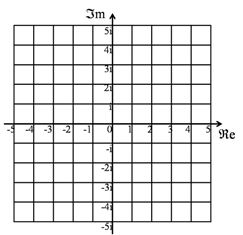The Argand Diagram is a geometric representation of Complex Numbers. The real number part is taken as a distance along the X-axis, the imaginary number part is taken as the distance along the Y-axis, giving a point in two dimensional space.

Addition of complex numbers can be thought of as vector addition, and multiplication is done by multiplying the lengths of the vectors, and adding the angles (taken positive in an anti-clockwise direction from the X-axis).

AbsoluteValue
CategoryMetaTopic
CauchySequence
CommonCoreDomains
CommonFactor
ComplexConjugate
DifferenceOfTwoSquares
DividingComplexNumbers
Divisor
DomainOfAFunction
FundamentalTheoremOfAlgebra
HolomorphicFunction
ImaginaryNumber
IsaacNewton
Logarithm
MathematicsTaxonomy
MultiplyingComplexNumbers
Polynomial
Quaternion
RootsOfPolynomials
SquareRoot
TypesOfNumber
NewtonsMethod
PolarRepresentationOfAComplexNumber
WhatIsATopic
BasinOfAttraction
PythagorasTheorem
RiemannSurface
RiemannZetaFunction
SubtractingComplexNumbers
ComplexPlane
MagnitudeOfAComplexNumber

## You are here

ArgandDiagram
ImaginaryNumber
RealNumber
Vectors
ComplexConjugate
ComplexPlane
DividingComplexNumbers
Euler
MultiplyingComplexNumbers
PolarRepresentationOfAComplexNumber
AlgebraicNumber
CauchySequence
ContinuedFraction
DedekindCut
IrrationalNumber
RationalNumber
ScalarProduct
SquareRoot
TranscendentalNumber